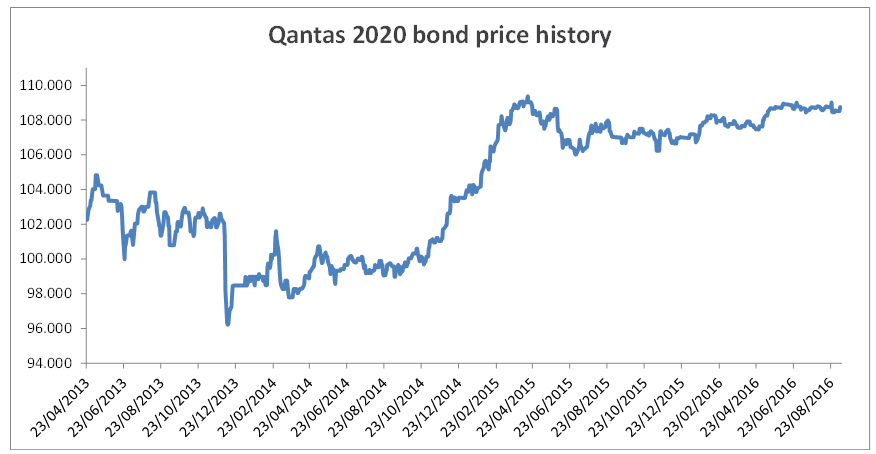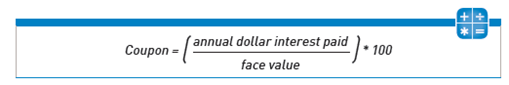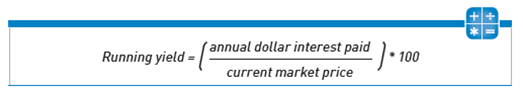Monday 12 September 2016 by Education (basics)

# Understanding bond yields — A piece of cake

## With investors hungry for higher returns, we examine the different measures of yield and how they are calculated – so you can have your cake and eat it tooThe yield is the expected return on an investment. The yield or rate of return can be described in a number of ways – we use the following Qantas fixed rate bond to illustrate yield concepts.

Issuer Description Maturity date Nominal yield Coupon type Frequency Yield to maturity Running yield Minimum investment Capital price
Qantas Airways Limited QAN-6.5%-27Apr20 27/04/2020 6.50% Fixed Semi annual 3.89% 5.98% \$50,000  108.723
Source: FIIG Securities
Pricing accurate as of 12 September 2016 but subject to change.

Below is a history of the Qantas 2020 bond ‘clean price’, which is the capital price excluding any accrued interest. You can see that the price of the bond has moved quite a lot over its life. It began at its \$100 face value but since has reached a high of \$109.36 and a low price of \$96.21.Source: FIIG Securities

Changes in yield, correspond to changes in a bond’s price, and understanding these movements can help investors construct and weight their portfolio to maximise returns. We explain this through three yield concepts: nominal yield, yield to maturity and running yield.

Nominal yield

The nominal yield, or coupon, is the return based on annual coupon payments as a percentage of the face value of the security. For a fixed rate bond, this does not change throughout the life of the security.For example, the Qantas 6.5% fixed rate bond will pay a coupon of \$6.5 a year based on a \$100 (face value) investment.

Coupons are periodical interest payments that an investor receives for holding a bond. Coupons can be fixed or floating. This means they are either a set interest rate determined at the time of issue of the security, or they can vary with respect to some form of floating benchmark, such as the bank bill swap rate (BBSW). Coupons are normally paid on either a quarterly, semi annual or annual basis.

Australian floating rate bonds generally pay a quarterly coupon while fixed rate bonds mostly pay a semi annual coupon. Fixed rate bond semi annual coupon payments are a flat annual coupon payment divided by two; there is no adjustment for the number of actual days in the coupon period. So in the instance of the Qantas fixed rate bond, if the coupon is 6.5% paid on a semi annual basis, the holder receives \$3.25 per \$100 of face value every six months.

The coupon rate may or may not represent the actual return the investor receives from the bond and this is determined by the purchase price of the bond. The purchase price can be at par (\$100), at a discount to face value (less than \$100), or at a premium to face value (more than \$100). So, depending on the purchase price of the bond, the actual return to the investor may be higher or lower than the coupon rate. This measure of return is known as yield to maturity.

Yield to Maturity

The yield to maturity refers to how much a security will earn if it is held to its maturity date. It is the annualised return based on all coupon payments plus face value or the market price if it was purchased on the secondary market. Yield to maturity includes any gain or loss if the purchase price was below or above the face value. For this reason, the yield to maturity is considered the most important variable of bond analysis as it provides a basis for comparison between different securities and other interest rate based products.

If a bond is trading at a discount, the yield to maturity will be the running yield plus the annualised capital gain between the current market price and \$100 face value, which is returned at maturity and adjusted for discounting or the time value of money.

However, if a bond is trading at a premium, the yield to maturity will be the running yield less the annualised capital loss being the difference between the current market price and \$100 face value, which is returned upon maturity and adjusted for discounting.

For example, the Qantas fixed rate bond currently has a nominal yield of 6.5%, a maturity of 27 April 2020 and is currently trading at a premium to face value at around \$108.72. If we calculate yield to maturity we find it is 3.89% compared to the coupon rate of 6.5%. This means that the effective return over the life of the security, if bought today, would be 3.89% taking into account the current premium price of the security.

Note that the calculation makes the assumption that all coupon payments can be reinvested at the yield to maturity rate.

All prices and yields are accurate as at 12 September 2016 and are a guide only, subject to market availability. FIIG does not make a market in these securities.

Running Yield

Another measure to compare the return of bonds is the running yield. Running yield uses the current price of a bond instead of its face value and represents the return an investor would expect if they purchased a bond and held it for a year. It is calculated by dividing the coupon by the market price as shown below.For example, if you purchase the Qantas bond for the current market price – also known as the capital price – of \$108.72 and this bond pays a coupon of 6.5% on the face value (\$100), you will receive a cashflow of \$6.5 a year. Given this return is achieved at a premium to face value of \$108.72, instead of \$100 face value, your actual return will be less than 6.5%.

Therefore, your running yield would be 5.98% (6.5/108.72 x 100 = 5.98). As the bond price increases, running yield decreases, and as the bond price decreases the running yield would increase. Likewise, if a fixed rate bond is trading at a discount, the running yield will be higher than the nominal yield.

> View all